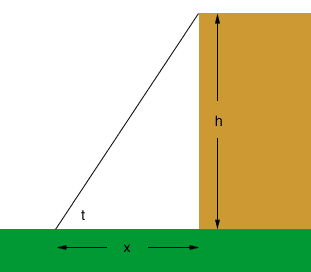Quandaries and Queries thank you for replying.....if you know the distance to the base and the angle to the top how can you determine the height thanks paul Hi Paul, This is an exercise in trigonometry. In the diagram below x is the distance to the base, t is the angle measurement and h is the unknown height.The trigonometric function that relates these variables is tan(t) = h/x so h = xtan(t). Penny